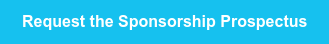<img height="1" width="1" style="display:none" alt="" src="https://www.facebook.com/tr?id=367542720414923&amp;ev=PageView&amp;noscript=1">10am - 1pm AEST

12pm - 3pm NZSTtrue true true true true true true true true true true true true true true true true true true true true true true true true true true true true true true true true true true true true true true true true true true true true true true true true true true true true true true true true true true true true true true true true true true true true true true true true true true true true true true true true true true true true true true true true true true true true true true true true true true true true true true true true true true true true true true true true true true true true true true true true true true true true true true true true true true true true true true true true true true true true true true true true true true true true true true true true true true true true true true true true true true true true true true true true true true true true true true true true true true true true true true true true true true true true true true true true true true true true true true true true true true true true true true true true true true true true true true true true true true true true true true true true true true true true true true true true true true true true true true true true true true true true true true true true true true true true true true true true true true true true true true true true true true true true true true true true